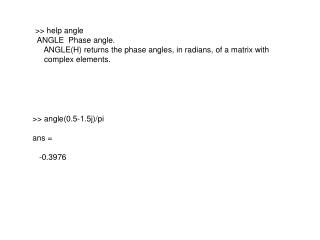DownloadDownload Presentation>> help angle ANGLE Phase angle.

# >> help angle ANGLE Phase angle.

Download Presentation## >> help angle ANGLE Phase angle.

- - - - - - - - - - - - - - - - - - - - - - - - - - - E N D - - - - - - - - - - - - - - - - - - - - - - - - - - -
##### Presentation Transcript

1. >> help angle ANGLE Phase angle. ANGLE(H) returns the phase angles, in radians, of a matrix with complex elements. >> angle(0.5-1.5j)/pi ans = -0.3976

2. >> b=1; a=poly([1,1,-1]) a = 1 -1 -1 1 >> [R,p,c]=residuez(b,a) R = 0.25000000000000 0.25000000000000 + 0.00000000093614i 0.50000000000000 - 0.00000000093614i p = -1.00000000000000 1.00000000000000 + 0.00000000374454i 1.00000000000000 - 0.00000000374454i c = []